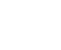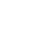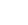# Multiset, Multiplicity and Multichoose: Simple Definition & Examples

Contents:

## 1. What is a Multiset?

A multiset in mathematics is a generalization of the concept of a set. It’s a collection of unordered numbers (or other elements), where every element x occurs a finite number of times.

The difference between sets and multisets is in how they address multiples: a set includes any number at most once, while a multiset allows for multiple instances of the same number. There is just one set with elements a and b, the set {a,b}, but there are many multisets: {a, b, b}, {a, a, b}, and {a, a, a, a, b, b} are just a few.

The number of multisets k long using n symbols is called n multichoose k, and it’s written as

## Multiplicity

The multiplicity of an element x in a multiset is just the number of times that element appears in the set. For instance, in the multiset {3, 3, 4, 5, 6} the element 3 has multiplicity 2. The elements 4, 5, and 6 all have multiplicity 1.

If we know the elements included and the multiplicity for each of them we know everything about a multiset. A multiset which includes just 4 with multiplicity 2, 5 with multiplicity 7 and 99 with multiplicity 2 can be written as {4, 4, 5, 5, 5, 5, 5, 99, 99}. Order doesn’t matter, so this is the same as {4, 99, 5, 4, 99, 5, 5, 5, 5 }

## The Multiset in Use

Multisets are important in both math and computer science. They help us keep track of elements in databases, and are the backbone of modern combinatorics.

One example of multisets is a listing of the prime factors of a number. The factors of 4 is {2,2}, and this is different than the prime factors of 2,{2}. The prime factors of 90, {3,3,5,2}, are different than the prime factors of 30: {3,5,2}

## 2. What is Multichoose?

Multichoose is a way to choose items, where n is the number of items to choose from and k is the sets of items to choose. For example, 10 multichoose 4 is the number of possible ways to choose a set of 4 items from a group of 10 different items. More technically, it is the number of multisets k long, made up of n symbols. Order is not important, and repeats are allowed.

For example, 4 multichoose 2 equals 10, because the possible number of two-item multisets for four elements {j, k, l, m} are {j, j}, {j, k}, {j, l}, {j, m}, {k, k}, {k, l}, {k, m}, {l, l}, {l, m} and {m, m}.

## Findingn multichoose k

For small sets, the easiest way is to just list all of the possibilities. For example:Is asking “how many multisets from the top value (2 items long) can be found from the bottom value (the set {1,2})”?
The solution is 3: {1,1}, {1,2}, {2,2}.

Solutions to multichoose problems can also be found by justifying the columns of Pascal’s triangle and moving the numbers up (Isaak, 2016; Bailer-Jones, 2017):The first few {n,k} solutions as found in Pascal’s triangle.

## Comparison to Binomial Coefficient

We write the multichoose coefficient with double parentheses:.

You may notice this equation is similar to the binomial coefficient:The two are very similar. The definitions for the two coefficients (from Arthur et. al, 2003) are:

• Binomial coefficient: the number of k-element subsets of {1,…,n}
• Multichoose coefficient: the number of k-element multisubsets of {1,…,n}.

The binomial coefficient tells us the number of ways we can pick k unordered outcomes from n possibilities. Repeats are not allowed in the binomial coefficient; each of the n possibilities can only be chosen once. In multichoose, repeats are allowed: we can choose multiple times from the same possibility.

## Stars and Bars

Sometimes multichoose problems are called stars and bars problems. That’s because one way of solving them involves imagining our k items are k stars and we have n – 1 boundary points separating the types. The way the stars and boundary points are placed define the problem.

For example, in our candy store problem we have 4 – 1 = three bars ||| separating the types (i.e. 1|2|3|4). Since order doesn’t matter in multichoose problems, we can order our candy selections any way that makes it convenient for keeping track of them. This involves always having the same items in the same order. Here we might first mark down any licorice chosen, then peppermint drops, then lemon drops, and finally truffles. In order, those are:

1. Licorice
2. Peppermint
3. Lemon
4. Truffles

So if we just choose a licorice, we can write that as ☆|||.
If we just choose a peppermint drop, make that |☆||.
Just a lemon drops would be ||☆|,
and just a truffle |||☆.

One licorice and one truffle would be written ☆|||☆.

Example question (Benjamin & Quinn, 2003): Use stars and bars to represent the following distribution of 10 candies to 4 children:

• Child 1 receives 4 candies,
• Child 2 receives 5 candies,
• Child 3 receives 0 candies,
• Child 4 receives 1 candy.

Solution:
☆☆☆☆|☆☆☆☆☆||☆

## References

Isaak (2016). Practical Bayesian Inference. Cambridge University Press.
Bailer-Jones (2017). Choose Multichoose. 47th SEICCGTC at FAU, March 2016. Retrieved October 8, 2016 from: http://www.lehigh.edu/~gi02/Boca16b.pdf
Benjamin & Quinn (2003). Proofs that Really Count: The Art of Combinatorial Proof. MAA.

CITE THIS AS:
Stephanie Glen. "Multiset, Multiplicity and Multichoose: Simple Definition & Examples" From StatisticsHowTo.com: Elementary Statistics for the rest of us! https://www.statisticshowto.com/multiset/
---------------------------------------------------------------------------Need help with a homework or test question? With Chegg Study, you can get step-by-step solutions to your questions from an expert in the field. Your first 30 minutes with a Chegg tutor is free!